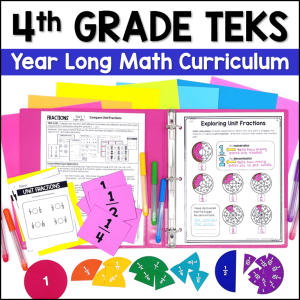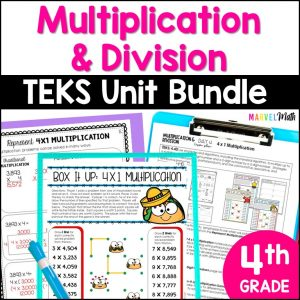Save 10% on your first order with code EXTRA10

# Multiplication & Division at the Beach

\$0.00187.25\$30.99
SKU: 3803968 Categories: ,

## Description

I hope you enjoy this resource to give your students meaningfully paired multiplication and division practice! Students will practice the more challenging multiplication facts to prepare them for the paired division page. 3rd graders can practice using related multiplication facts to solve division problems and 4th & 5th graders can practice long division while dreaming of the beach.??

?This resource comes in Color and B&W.

Print & Laminate the Color Pages for a fun Math Station.

Print the B&W pages to use as a stand-alone printable.

?Keys are included for teacher use and also to keep at stations so that they are self-checking.

TEKS Alignment:

3.4(F)

Recall facts to multiply up to a 10 by 10 with automaticity and recall the corresponding division facts

3.4(J)

Determine a quotient using the relationship between multiplication and division

4.4(D)

Use strategies and algorithms, including the standard algorithm, to multiply up to a four-digit number by a one-digit number and to multiply a two-digit number by a two-digit number

4.4 (F)

Use strategies and algorithms, including the standard algorithm, to divide up to a four-digit dividend by a one-digit divisor

5.3(C)

Solve with proficiency for quotients of up to a four-digit dividend by a two-digit divsor using strategies and the standard algorithm